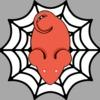## Welcome to the Treehouse Community

The Treehouse Community is a meeting place for developers, designers, and programmers of all backgrounds and skill levels to get support. Collaborate here on code errors or bugs that you need feedback on, or asking for an extra set of eyes on your latest project. Join thousands of Treehouse students and alumni in the community today. (Note: Only Treehouse students can comment or ask questions, but non-students are welcome to browse our conversations.)

### Looking to learn something new?

Treehouse offers a seven day free trial for new students. Get access to thousands of hours of content and a supportive community. Start your free trial today.# Medal will not display in 'Results' message? Code is correct yet still leaves 'empty space' when should interpolate?

Coded proper conditional statement, I tried both 'let rank;' as well as 'let rank = ''; and still comes up blank. \${rank} is placed properly, ie - <h2> You earned the \${rank} medal!</h2>`;

Not sure what the issue is?Looks like you haven't assigned a value to rank when you used it in for the totalScore variable. So when the program is at that line, rank is still = "";

```let rank = "";
.
.
.
const totalScore = `<h1> You got \${correct} out of 5 correct. </h1>;
<h2> You earned the \${rank} medal!</h2>`; // <-- here rank is still = ""
```

Since later in the program you do give a value to rank, like so:

```if ( correct === 5 ) {
rank = "Gold";
} else if ( correct >= 3 ) {
rank = "Silver";
} else if ( correct >= 1 ) {
rank = "Bronze";
} else {
rank = "NONE! Go watch the series again and come back! OR read the Manga FOO!";
}
```

You may want to rearrange the flow of these parts so that they pass along the information in the desired manner.Got it! Thanks so much! Also my set up with document.querySelector was a little goofy when selecting the 'main' element. I tried to explore and do it another way and it worked half way, but made the adjustment and works great. Thanks for your help! #learningSure, no problem!

```/*
- When quiz begins, no answers are correct
*/
let correct = 0;

//2. Store the rank of a player
let rank = "";

// 3. Select the <main> HTML element

/*
4. Ask at least 5 questions
- Store each answer in a variable
- Keep track of the number of correct answers
*/
const q1 = prompt("What is the demon's name that kills the fire Hashira?");
if ( q1.toUpperCase() === 'AKAZA' ) {
correct += 1;
}
console.log(correct);
let q2 = prompt("What is Tanjiro's first trainer's name? (Proper spelling)");
if ( q2.toUpperCase() === 'UROKODAKI' ) {
correct += 1;
}
console.log(correct);
let q3 = prompt("How many arms does Sasumaru (ball throwing demon) truly have?")
if ( q3 === `6`) {
correct += 1;
}
console.log(correct);
let q4 = prompt("What color does Tanjiro's sword turn?");
if ( q4.toUpperCase() === 'BLACK' ) {
correct += 1;
}
console.log(correct);
let q5 = prompt("This one's a toughie! What is Tanjiro's flame form really called? Good luck!");
if ( q5.toUpperCase() === 'HINOKAMI KAGURA' ) {
correct += 1;
}
console.log(correct);

const totalScore = `<h1> You got \${correct} out of 5 correct. </h1>;
<h2> You earned the \${rank} medal!</h2>`;

/*
5. Rank player based on number of correct answers
- 5 correct = Gold
- 3-4 correct = Silver
- 1-2 correct = Bronze
- 0 correct = No crown
*/

if ( correct === 5 ) {
rank = "Gold";
} else if ( correct >= 3 ) {
rank = "Silver";
} else if ( correct >= 1 ) {
rank = "Bronze";
} else {
rank = "NONE! Go watch the series again and come back! OR read the Manga FOO!";
}

// 6. Output results to the <main> element
document.querySelector('main').innerHTML = totalScore;
```